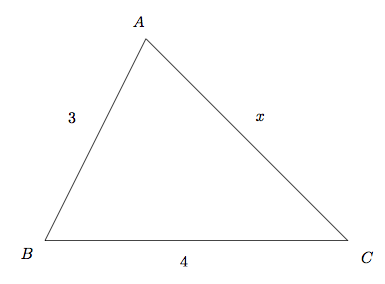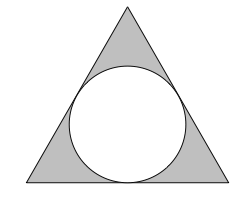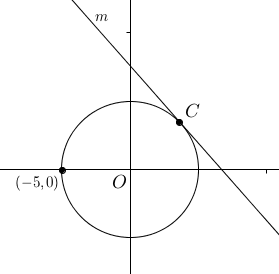# 7 Forgotten SAT Math Concepts That Could Prevent You From an 800

#### 1. Triangle Inequality Theorem

Two sides of a triangle must add up to be greater than the third side.

Example: In $$\triangle ABC$$, $$AB$$ has a length of $$3$$ and $$BC$$ has a length of $$4$$. How many integer values are possible for the length of $$AC$$?

Solution: The golden rule of any geometry problem is to draw a picture:Based on the theorem, we can come up with

$3 + 4 \gt x$ $3 + x \gt 4$ $4 + x \gt 3$

Which simplifies to

$7 \gt x$ $x \gt 1$ $x \gt -1$

From these inequalities, only the first two matter since the third is always taken care of by the first two. Therefore, $$1 \lt x \lt 7$$, and there are 5 integer values of $$x$$.

#### 2. Absolute Value

Absolute value is a way of specifying margin of error. $$\left|x - 5\right| \lt 7$$ means that $$x$$ has to be within $$7$$ units of $$5$$.

Example: A manufacturer of cookies tests the weight of its cookie packages to ensure consistency in the product. A package of cookies must weigh between $$16$$ ounces and $$18$$ ounces as it comes out of production. If $$w$$ is the weight of an accepted cookie package, then which of the following inequalities correctly expresses all possible values for $$w$$?

A) $$\left|w + 17\right| \lt 1$$
B) $$\left|w - 17\right| \gt 1$$
C) $$\left|w + 17\right| \gt 1$$
D) $$\left|w - 17\right| \lt 1$$

Solution: Notice that $$16 \lt w \lt 18$$. That means $$w$$ must be within $$1$$ ounce of $$17$$. Hence, the answer is D. Notice that $$\left|w - 17\right|$$ is how far off the weight is from $$17$$ ("margin of error"), and this margin of error must be less than $$1$$.

#### 3. Area of an Equilateral Triangle

Area of equilateral triangle $$= \dfrac{\sqrt{3}}{4}(\text{side})^2$$

Example: What is the area of an equilateral triangle of side length $$4$$?

A) $$2$$
B) $$2\sqrt{3}$$
C) $$4$$
D) $$4\sqrt{3}$$

Solution: Using the formula (which can easily be derived by using $$30-60-90$$ triangles), we get

$\text{Area} = \dfrac{\sqrt{3}}{4}(4)^2 = 4\sqrt{3}$

#### 4. Semiannual Compound Interest

Interest compounded semianually means that interest is earned twice a year, with each deposit at half the quoted yearly interest rate. For example, if a bank account earns 7% interest compounded semiannually, 3.5% would be deposited half a year in, and then another 3.5% would be deposited a year in. Keep in mind this second 3.5% deposit is on the total amount in the bank account, which includes the first interest deposit. Therefore, you earn more money with interest compounded semiannually than with interest compounded annually.

Example: Frank deposits $1,000 into a bank account that earns 5% interest compounded semianually. Which of the following is closest to the total amount in the account after 6 years? A)$1,160
B) $1,340 C)$1,345
D) $1,796 Solution: Interest is earned $$6\times 2 = 12$$ times. $\text{Total Amount} = \1,000(1.025)^{12} \approx \1,345$ The answer is C. #### 5. Inequalities The sign must be reversed if you multiply or divide by a negative at any point. Example: $-15n \leq -5(n - 4)$ Which of the following represents the solutions to the inequality shown above? A) $$n \leq -2$$ B) $$n \geq -2$$ C) $$n \leq 2$$ D) $$n \geq 2$$ Solution: $-15n \leq -5(n - 4)$ $-15n \leq -5n + 20$ $-10n \leq 20$ To isolate $$n$$, we now have to divide both sides by $$-10$$. Because we're dividing by a negative number, we have to reverse the sign. $n \geq -2$ The answer is B. #### 6. Direct and Indirect Proportion Direct proportion: $$y = kx$$, where $$k$$ is a constant (a.k.a a number like 5) Indirect proportion: $$xy = k$$ or $$y = \dfrac{k}{x}$$, where $$k$$ is a constant Example: A stone falls from a cliff. The distance it falls is directly proportional to the square of the time it has been falling. The stone falls $$19.6$$ meters after $$2$$ seconds. How far does it fall after $$3$$ seconds? Solution: Let's use the direct proportion equation: $d = kt^2$ It's important that we use $$t^2$$ instead of $$t$$ because as the problem specifies, the distance if proportional to the square of the time. Now let's solve for $$k$$: $19.6 = k \cdot (2)^2$ $19.6 = 4k$ $k = 4.9$ So now our equation becomes $d = 4.9t^2$ And when $$t = 3$$, $d = 4.9\cdot (3)^2$ $d = \boxed{44.1}$ #### 7. Radius drawn to a Tangent A radius drawn to a tangent of a circle is always perpendicular to that tangent. Example:In the figure above, the center of the circle is point $$B$$, and $$\overline{AC}$$ is tangent to the circle at point $$D$$. If $$AD = 2$$ and $$DC = 5$$, how much greater is the area of $$\triangle DBC$$ than the area of $$\triangle ABD$$? A) $$2$$ B) $$5$$ C) $$6$$ D) $$\text{It cannot be determined from the information given.}$$ Solution: Given the above rule, both $$\angle BDA$$ and $$\angle BDC$$ are right angles, making it easy to find the areas of the two triangles: $\text{Area of } \triangle DBC = \dfrac{5\cdot 4}{2} = 10$ $\text{Area of } \triangle ABD = \dfrac{2\cdot 4}{2} = 4$ So the difference between the two areas is 6, answer C. #### Your Turn: Practice Questions 1. For how many different integers $$x$$, with $$2 \lt x \lt 7$$, is there a triangle with sides of length $$2, 7,$$ and $$x$$? A) One B) Two C) Three D) Four 2. Rolls of tapes must be made to a certain length. They must contain enough tape to cover between $$400$$ feet and $$410$$ feet. If $$l$$ is the length of a roll of tape that meets the requirement, which of the following inequalities expresses the possible values for $$l$$? A) $$\left|l - 400\right| \lt 10$$ B) $$\left|l - 405\right| \gt 5$$ C) $$\left|l + 405\right| \lt 5$$ D) $$\left|l - 405\right| \lt 5$$ 3.In the figure shown above, a circle is inscribed inside an equilateral triangle with a side length of $$6$$. What is the area of the shaded region? (Hint: You'll need to use the $$30-60-90$$ triangle relationship.) A) $$6\sqrt{3} - 3\pi$$ B) $$6\sqrt{3} - 6\pi$$ C) $$9\sqrt{3} - 3\pi$$ D) $$9\sqrt{3} - 6\pi$$ 4. Melanie leaves$500 in a bank account that earns 8% interest compounded annually for 10 years. To the nearest dollar, how much more money would she have earned over the 10 year period if the interest had been compounded semiannually instead of annually?

A) 16
B) 18
C) 20
D) 25
5. If $$2m - 3 \geq 17$$ and $$-3n + 5 \geq -7$$, what is the minimum possible value of $$m - n$$?
6. $$y$$ is inversely proportional to $$x$$ and $$y = 8$$ when $$x = 3$$. What is the value of $$y$$ when $$x = 4$$?

A) $$5$$
B) $$6$$
C) $$7$$
D) $$8$$
7.The coordinates $$(x, y)$$ of each point on the circle above satisfy the equation $$x^2 + y^2 = 25$$. Line $$l$$ is tangent to the circle at point $$C$$. If the $$x$$-coordinate of point $$C$$ is $$3$$, what is the slope of $$l?$$

7. $$-\dfrac{3}{4}$$